Test: Profit & Loss- 3

# Test: Profit & Loss- 3

Test Description

## 15 Questions MCQ Test Quantitative Aptitude (Quant) | Test: Profit & Loss- 3

Test: Profit & Loss- 3 for Quant 2022 is part of Quantitative Aptitude (Quant) preparation. The Test: Profit & Loss- 3 questions and answers have been prepared according to the Quant exam syllabus.The Test: Profit & Loss- 3 MCQs are made for Quant 2022 Exam. Find important definitions, questions, notes, meanings, examples, exercises, MCQs and online tests for Test: Profit & Loss- 3 below.
Solutions of Test: Profit & Loss- 3 questions in English are available as part of our Quantitative Aptitude (Quant) for Quant & Test: Profit & Loss- 3 solutions in Hindi for Quantitative Aptitude (Quant) course. Download more important topics, notes, lectures and mock test series for Quant Exam by signing up for free. Attempt Test: Profit & Loss- 3 | 15 questions in 30 minutes | Mock test for Quant preparation | Free important questions MCQ to study Quantitative Aptitude (Quant) for Quant Exam | Download free PDF with solutions
 1 Crore+ students have signed up on EduRev. Have you?
Test: Profit & Loss- 3 - Question 1

### An article is bought for Rs 600 and sold for Rs 750. What is the profit percentage?

Detailed Solution for Test: Profit & Loss- 3 - Question 1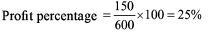Test: Profit & Loss- 3 - Question 2

### The profit obtained by selling a book for Rs 56 is the same as the loss obtained by selling this book for Rs 42. What is the cost price of the book?

Detailed Solution for Test: Profit & Loss- 3 - Question 2

Let us assume that the cost price = Rs X

56 - x = x - 42

2x = 98

Hence, x = 49

Test: Profit & Loss- 3 - Question 3

### By selling a CD for Rs 150, a shop owner lost 1/16 of what it costs. What is the cost price of CD?

Detailed Solution for Test: Profit & Loss- 3 - Question 3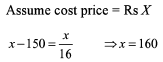Test: Profit & Loss- 3 - Question 4

A man sold an article at a profit of 10%. If he had charged Rs 45 more his profit percentage would have been 25%. What is the cost price?

Detailed Solution for Test: Profit & Loss- 3 - Question 4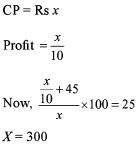Test: Profit & Loss- 3 - Question 5

A man buys 200 oranges for Rs 10. How many oranges a rupee can he sell so that his profit percentage is 25%?

Detailed Solution for Test: Profit & Loss- 3 - Question 5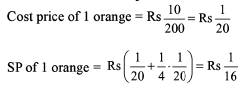So, he should sell 16 oranges in a rupee to make a profit of 25%.

Test: Profit & Loss- 3 - Question 6

By selling an article for Rs 360, the loss incurred is 10%. At what minimum price should he sell that article to avoid loss?

Detailed Solution for Test: Profit & Loss- 3 - Question 6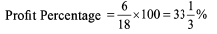Test: Profit & Loss- 3 - Question 7

The owner of Book Point does not get either profit or loss by selling 15 books for Rs 225. How many books should he sell for the same amount to gain 25% profit?

Detailed Solution for Test: Profit & Loss- 3 - Question 7

CP of 1 book = Rs 15 SP of 1 book in order to gain 25% profit = Rs 18.75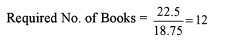Test: Profit & Loss- 3 - Question 8

CP of 12 apples is equal to the SP of 9 apples and the discount on 10 apples is equal to the profit on 5 apples. What is the percentage difference between the CP and SP of apples?

Detailed Solution for Test: Profit & Loss- 3 - Question 8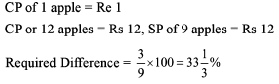Test: Profit & Loss- 3 - Question 9

A shopkeeper professes to sell his articles at CP but gives only N grams in the place of 1000 gm. If his profit percentage is 100%, what is the value of N?

Detailed Solution for Test: Profit & Loss- 3 - Question 9

B
(error÷1000-error)×100=1
0
(X/1000-x)×100= 100
100x = 100×(1000-x)

100x = 100000-100x

200x = 100000

X = 100000/200

X = 500

so error is 500 then N= 1000-500=500

Test: Profit & Loss- 3 - Question 10

A car is sold for Rs 2,400 at a profit of 20%. What is the CP?

Detailed Solution for Test: Profit & Loss- 3 - Question 10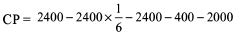Test: Profit & Loss- 3 - Question 11

A car is sold for Rs 2,400 at a profit of 20% over SR What is the actual profit percentage?

Detailed Solution for Test: Profit & Loss- 3 - Question 11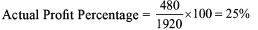Test: Profit & Loss- 3 - Question 12

Sharat sells some articles to Chandra at a profit of 20%. Chandra now sells this article to Mayank at a loss of 30% and Mayank sells this article at a profit of 20%. If CP of Chandra is Rs 150, then what is the SP of Mayank?

Detailed Solution for Test: Profit & Loss- 3 - Question 12

CP of Mayank = CP of Chandra * 0.7 = Rs 150 x 0.7 = Rs 105 SP of Mayank = Rs 126

Test: Profit & Loss- 3 - Question 13

A shopkeeper wants to earn a profit of 20% and at the same time, the minimum discount which he wants to offer is 25%. What should be the minimum percentage mark-up over CP?

Detailed Solution for Test: Profit & Loss- 3 - Question 13

Let CP = Rs 100

SP = Rs 120

MP = x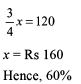Test: Profit & Loss- 3 - Question 14

An article when sold at 10% discount on the marked price gave a profit of Rs 70. What is the CP?

Detailed Solution for Test: Profit & Loss- 3 - Question 14

Without knowing the profit percentage, we cannot determine the CR

Test: Profit & Loss- 3 - Question 15

A milkman buys two cows for Rs 750. He sells first cow at a profit of 22% and the second cow at a loss of 8%. What is the SP of second cow if in the whole transaction there is no profit no loss?

Detailed Solution for Test: Profit & Loss- 3 - Question 15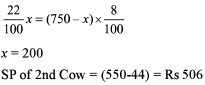## Quantitative Aptitude (Quant)

163 videos|152 docs|131 tests
 Use Code STAYHOME200 and get INR 200 additional OFF Use Coupon Code
Information about Test: Profit & Loss- 3 Page
In this test you can find the Exam questions for Test: Profit & Loss- 3 solved & explained in the simplest way possible. Besides giving Questions and answers for Test: Profit & Loss- 3, EduRev gives you an ample number of Online tests for practice

## Quantitative Aptitude (Quant)

163 videos|152 docs|131 tests